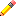LoopsSo far we have introduced a couple of EBNF operators that deal with looping. We have the + positive operator, which matches the preceding symbol one (1) or more times, as well as the Kleene star * which matches the preceding symbol zero (0) or more times.

Taking this further, we may want to have a generalized loop operator. To some this may seem to be a case of overkill. Yet there are grammars that are impractical and cumbersome, if not impossible, for the basic EBNF iteration syntax to specify. Examples:A file name may have a maximum of 255 characters only.A specific bitmap file format has exactly 4096 RGB color information.A 32 bit binary string (1..32 1s or 0s).

Other than the Kleene star *, the Positive closure +, and the optional !, a more flexible mechanism for looping is provided for by the framework.

 Loop Constructs repeat_p (n) [p] Repeat p exactly n times repeat_p (n1, n2) [p] Repeat p at least n1 times and at most n2 times repeat_p (n, more) [p] Repeat p at least n times, continuing until p fails or the input is consumed

Using the repeat_p parser, we can now write our examples above:

A file name with a maximum of 255 characters:

```    valid_fname_chars = /*..*/;
filename = repeat_p(1, 255)[valid_fname_chars];```

A specific bitmap file format which has exactly 4096 RGB color information:

```    uint_parser<unsigned, 16, 6, 6> rgb_p;
bitmap = repeat_p(4096)[rgb_p];```

As for the 32 bit binary string (1..32 1s or 0s), of course we could have easily used the bin_p numeric parser instead. For the sake of demonstration however:

`    bin32 = lexeme_d[repeat_p(1, 32)[ch_p('1') | '0']];`Loop parsers are run-time parametric.

The Loop parsers can be dynamic. Consider the parsing of a binary file of Pascal-style length prefixed string, where the first byte determines the length of the incoming string. Here's a sample input:

 11 h e l l o _ w o r l d

This trivial example cannot be practically defined in traditional EBNF. Although some EBNF syntax allow more powerful repetition constructs other than the Kleene star, we are still limited to parsing fixed strings. The nature of EBNF forces the repetition factor to be a constant. On the other hand, Spirit allows the repetition factor to be variable at run time. We could write a grammar that accepts the input string above:

```    int c;
r = anychar_p[assign_a(c)] >> repeat_p(boost::ref(c))[anychar_p];```

The expression

`    anychar_p[assign_a(c)]`

extracts the first character from the input and puts it in c. What is interesting is that in addition to constants, we can also use variables as parameters to repeat_p, as demonstrated in

`    repeat_p(boost::ref(c))[anychar_p]`

Notice that boost::ref is used to reference the integer c. This usage of repeat_p makes the parser defer the evaluation of the repetition factor until it is actually needed. Continuing our example, since the value 11 is already extracted from the input, repeat_p is is now expected to loop exactly 11 times.